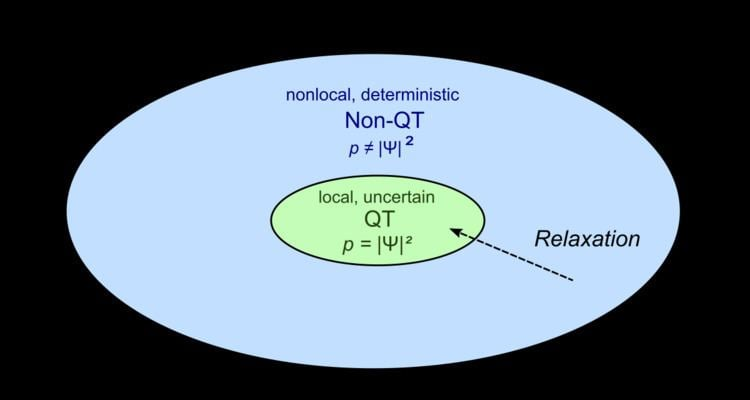# Quantum non equilibrium

Updated on
Covid-19Quantum non-equilibrium is a concept within stochastic formulations of the De Broglie–Bohm theory of quantum physics.

## Contents

In the Copenhagen interpretation, that is, the most widely used interpretation of quantum mechanics, the Born rule ρ ( x , t ) = | ψ ( x , t ) | 2 defines that ρ , the probability density function of a particle (that is, the probability to find the particle in the differential volume d 3 x at time t) equals the absolute square of the wave function ψ , and it constitutes one of the fundamental axioms of the theory.

This is not the case for the De Broglie–Bohm theory, where the Born rule is not a basic law. Rather, in this theory the link between the probability density and the wave function has the status of a hypothesis, called the quantum equilibrium hypothesis, which is additional to the basic principles governing the wave function, the dynamics of the quantum particles and the Schrödinger equation. (For mathematical details, refer to the derivation by Peter R. Holland.)

Accordingly, quantum non-equilibrium describes a state of affairs where the Born rule is not fulfilled; that is, the probability to find the particle in the differential volume d 3 x at time t is unequal to | ψ ( x , t ) | 2 .

Recent advances in investigations into properties of quantum non-equilibrium states have been performed mainly by theoretical physicist Antony Valentini, and earlier steps in this direction were undertaken by David Bohm, Jean-Pierre Vigier, Basil Hiley and Peter R. Holland. The existence of quantum non-equilibrium states has not been verified experimentally; quantum non-equilibrium is so far a theoretical construct. The relevance of quantum non-equilibrium states to physics lies in the fact that they can lead to different predictions for results of experiments, depending on whether the De Broglie–Bohm theory in its stochastic form or the Copenhagen interpretation is assumed to describe reality. (The Copenhagen interpretation, which stipulates the Born rule a priori, does not foresee the existence of quantum non-equilibrium states at all.) That is, properties of quantum non-equilibrium can make certain classes of Bohmian theories falsifiable according to the criterion of Karl Popper.

In practice, when performing Bohmian mechanics computations in quantum chemistry, the quantum equilibrium hypothesis is simply considered to be fulfilled, in order to predict system behaviour and the outcome of measurements.

## Relaxation to equilibrium

The causal interpretation of quantum mechanics has been set up by de Broglie and Bohm as a causal, deterministic model, and it was extended later by Bohm, Vigier, Hiley, Valentini and others to include stochastic properties.

Bohm and other physicists, including Valentini, view the Born rule linking R to the probability density function ρ = R 2 as representing not a basic law, but rather as constituting a result of a system having reached quantum equilibrium during the course of the time development under the Schrödinger equation. It can be shown that, once an equilibrium has been reached, the system remains in such equilibrium over the course of its further evolution: this follows from the continuity equation associated with the Schrödinger evolution of ψ . However, it is less straightforward to demonstrate whether and how such an equilibrium is reached in the first place.

In 1991, Valentini provided indications for deriving the quantum equilibrium hypothesis which states that ρ ( X , t ) = | ψ ( X , t ) | 2 in the framework of the pilot wave theory. (Here, X stands for the collective coordinates of the system in configuration space). Valentini showed that the relaxation ρ ( X , t ) | ψ ( X , t ) | 2 may be accounted for by a H-theorem constructed in analogy to the Boltzmann H-theorem of statistical mechanics.

Valentini's derivation of the quantum equilibrium hypothesis was criticized by Detlef Dürr and co-workers in 1992, and the derivation of the quantum equilibrium hypothesis has remained a topic of active investigation.

Numerical simulations demonstrate a tendency for Born rule distributions to arise spontaneously at short time scales.

## Predicted properties of quantum non-equilibrium

Valentini showed that his expansion of the De Broglie–Bohm theory would allow “signal nonlocality” for non-equilibrium cases in which ρ ( x , y , z , t ) | ψ ( x , y , z , t ) | 2 , thereby violating the assumption that signals cannot travel faster than the speed of light.

Valentini furthermore showed that an ensemble of particles with known wave function and known nonequilibrium distribution could be used to perform, on another system, measurements that violate the uncertainty principle.

These predictions differ from predictions that would result from approaching the same physical situation by means of the Copenhagen interpretation and therefore would in principle make the predictions of this theory accessible to experimental study. As it is unknown whether or how quantum non-equilibrium states can be produced, it is difficult or impossible to perform such experiments.

However, also the hypothesis of quantum non-equilibrium big bang gives rise to quantitative predictions for nonequilibrium deviations from quantum theory which appear to be more easily accessible to observation.

## References

Similar Topics
Accident (2012 film)
Rohit Brijnath
Holly Holm
Topics

 B i Link H2
 L